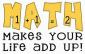# help: graph of x^(3/2) + y^(3/2) = 4^(3/2)

2 posts / 0 new
Juny Geraldizo ...help: graph of x^(3/2) + y^(3/2) = 4^(3/2)

can anyone help me how to graph this equation

x^(3/2) + y^(3/2) = 4^(3/2)

thanks for those who will response

Jhun VertBy inspection, the graph cannot exist at quadrants where x and y are negative because when x is negative x3/2 is imaginary, the same true to y. We can therefore see that the graph will only lie on the 1st quadrant

Try point plotting and rewrite the equation into y = (8 - x1.5)2/3

 x 0 1 2 3 4 y 4 3.66 2.99 1.99 0

For finer graph, you may increment by 0.5 instead of by 1. Hint: If you are using a calculator with table capability you can tabulate the data above. For CASIO fx-991ES use MODE 7:TABLE

• Mathematics inside the configured delimiters is rendered by MathJax. The default math delimiters are $$...$$ and $...$ for displayed mathematics, and $...$ and $...$ for in-line mathematics.